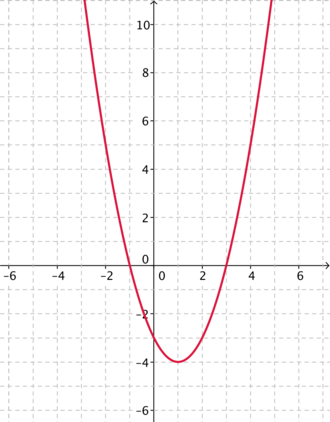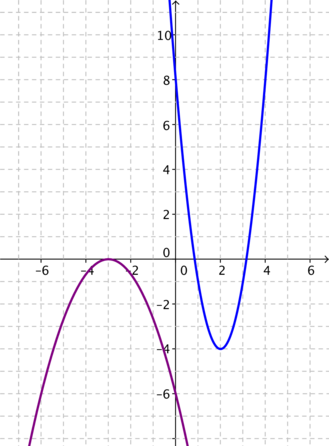#### You may also likeThis comes in two parts, with the first being less fiendish than the second. Itâ€™s great for practising both quadratics and laws of indices, and you can get a lot from making sure that you find all the solutions. For a real challenge (requiring a bit more knowledge), you could consider finding the complex solutions.### Discriminating

You're invited to decide whether statements about the number of solutions of a quadratic equation are always, sometimes or never true.This will encourage you to think about whether all quadratics can be factorised and to develop a better understanding of the effect that changing the coefficients has on the factorised form.

# Name That Graph

##### Age 16 to 18Challenge LevelThis resource is from Underground Mathematics.

Take a look at the parabola below.Can you find an equation for this parabola?

• In how many ways can you choose to show this equation?
• Which format did you initially choose and why?

What is the same and what is different about your approach to finding an equation of the two parabolas below, compared to the example above?• What properties do the three parabolas have in common?
• Is there an approach to finding the equation that works for all three of these parabolas, and indeed for any parabola you could be given?
• Which approach to finding the equation was the most efficient? Is it the same approach for each example?

This is an Underground Mathematics resource.

Underground Mathematics is hosted by Cambridge Mathematics. The project was originally funded by a grant from the UK Department for Education to provide free web-based resources that support the teaching and learning of post-16 mathematics.

Visit the site at undergroundmathematics.org to find more resources, which also offer suggestions, solutions and teacher notes to help with their use in the classroom.